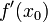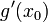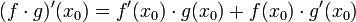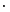# Product rule for differentiation of dot product

Specific point, named functions Suppose$f$ and$g$ are$n$-dimensional. vector-valued functions of a single variable and$x_0$ is a point in the domain such that$\! f'(x_0)$ and$\! g'(x_0)$ both exist (again, as$n$-dimensional vectors). Then$(f \cdot g)'(x_0) = f'(x_0) \cdot g(x_0) + f(x_0) \cdot g'(x_0)$ where$\cdot$ denotes the dot product.